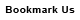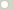# Metric conversions

ConvertPlus.Com provides easy to use and convenient metric conversions for over 1,000 various units and measurements, including weight, length, volume, area and more.

To do your metric conversion go to the conversion page for your measure (e.g. weight or area), type your value in the box near the unit you are conversing from (e.g. foot) and click "convert" button.

The value you have typed gets instantly converted to all other possible units! You only need to read you answer near the unit you need (e.g. meter)

The full list of measurements available for conversions (including metric conversions) is available on the main conversion page of our site.# Metric System Principles

The metric system was devised in France in the 18th century. The goal was to replace the chaotic collection of units being in use with a system based on one standard and decimal factors.
The standard for length was created by dividing the quarter of the Earth diameter by ten millions. The resulting length was named meter. The definition of meter changed several times since to make it more precise. The latest and the most precise definition of meter is "the distance light travels in a vacuum in 1/299,792,458 second". The standards for other measurements in metric system were set in a similar way.
The metric system or International System of Units (SI) is based on seven base units for seven base quantities that are mutually independent. The quantites are: length (meter), mass (kilogram), time (second), electric current (ampere), thermodynamic temperature (kelvin), amount of substance (mole) and luminous intensity (candela). All other units are derived from the base ones.
All units of a particular measurement are consctructed from the corresponding base unit by adding some metric prefix. The table of metric prefixes useful for metric conversions is provided below.# Metric Prefixes

Metric prefixes are pretty easy to understand and very handy for metric conversions. You don't have to know the nature of a unit to convert, for example, from kilo-unit to mega-unit. All metric prefixes are powers of 10. The most commonly used prefixes are highlighted in the table.

 Prexis Symbol Factor yotta Y 1024 = 1,000,000,000,000,000,000,000,000 zetta Z 1021 = 1,000,000,000,000,000,000,000 exa E 1018 = 1,000,000,000,000,000,000 peta P 1015 = 1,000,000,000,000,000 tera T 1012 = 1,000,000,000,000 giga G 109 = 1,000,000,000 mega M 106 = 1,000,000 kilo k 103 = 1,000 hecto h 102 = 100 deka da 101 = 10 deci d 10-1 = 0.1 centi c 10-2 = 0.01 milli m 10-3 = 0.001 micro µ 10-6 = 0.000,001 nano n 10-9 = 0.000,000,001 pico p 10-12 = 0,000,000,000,001 femto f 10-15 = 0.000,000,000,000,001 atto a 10-18 = 0.000,000,000,000,000,001 zepto z 10-21 = 0.000,000,000,000,000,000,001 yocto y 10-24 = 0.000,000,000,000,000,000,000,001

Most people even in the countries where metric system is used only know the most important metric prefixes like 'kilo' and 'milli'. They are very handy for understanding metric conversions. The prefixes like 'zepto' or 'yotta' are very specific and used mostly in science.ConversionsAreaCapacity and VolumeCircular measureComputer storageCurrencyDistance and LengthEnergy and WorkFuel ConsumptionMass and WeightPowerPressureSpeedTemperatureTimeTorqueSearchLink to us